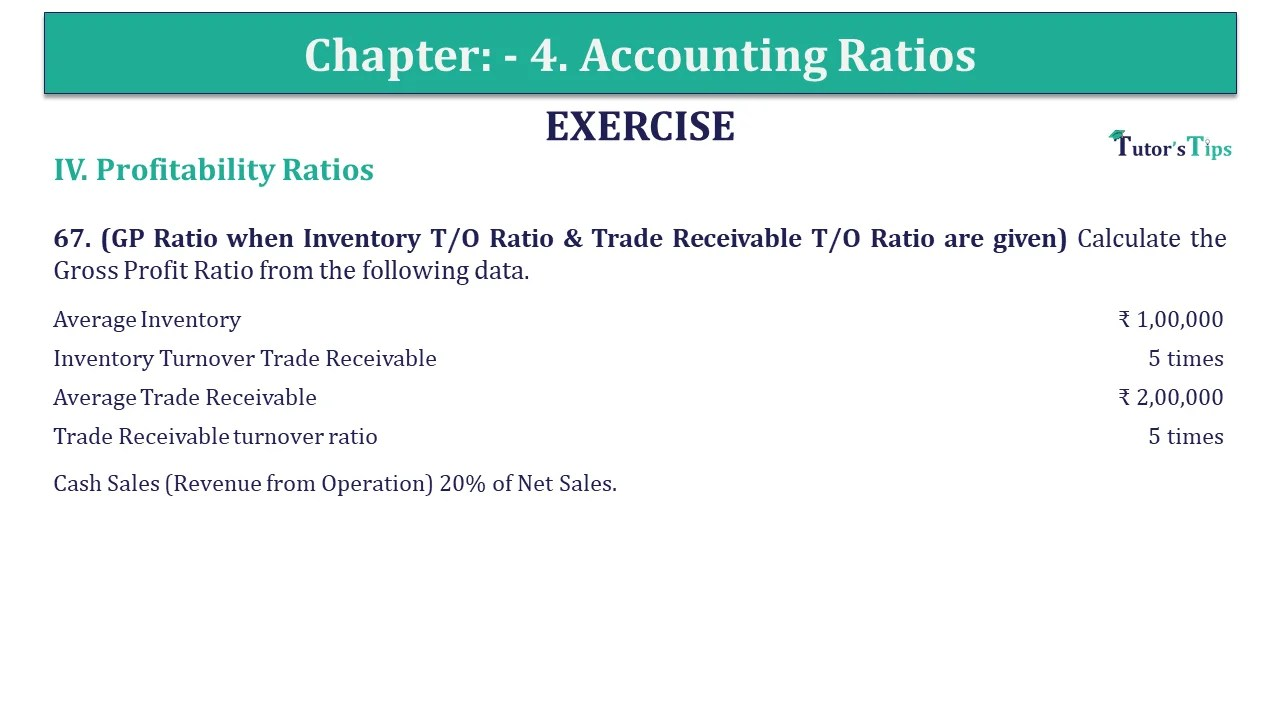# Question 67 Chapter 4 of +2-B – USHA Publication 12 ClassQ-67- CH-4 Book 2 - Usha Pub. +2 Book 2020 - Solution

Question 67 Chapter 4 of +2-B

67. (GP Ratio when Inventory T/O Ratio & Trade Receivable T/O Ratio are given) Calculate the Gross Profit Ratio from the following data.

 Average Inventory ₹ 1,00,000 Inventory Turnover Trade Receivable 5 times Average Trade Receivable ₹ 2,00,000 Trade Receivable turnover ratio 5 times

Cash Sales (Revenue from Operation) 20% of Net Sales.

## The solution of Question 67 Chapter 4 of +2-B: –

 Cost of goods sold = Average Inventory x Inventory Turnover Ratio = ₹ 1,00,000 x 5 = ₹ 5,00,000 Net Credit Sales = Average Trade Receivable x Trade Receivable turnover ratio Sales = ₹ 2,00,000 x 5 = ₹ 10,00,000
 Total Sales = 100 X ₹ 10,00,000 800 = ₹ 12,50,000 Gross Profit = Net Sales – Cost of goods sold = ₹ 12,50,000 – ₹ 5,00,000 = ₹ 7,50,000

 Gross Profit Ratio = Gross Profit X 100 Sales
 = ₹ 7,50,000 X ₹ 1,50,000 ₹ 12,50,000 = 60%

Also, Check out the solved question of previous Chapters: –